# 🎮 半小时开发3D博饼小游戏 | 文末在线玩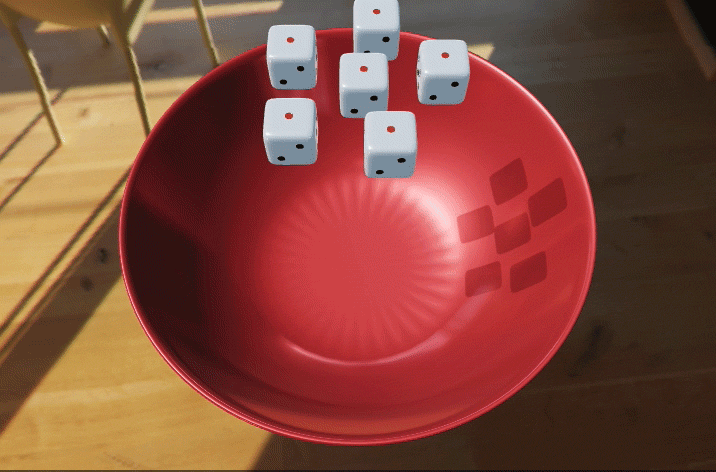# step.1 准备工作

## 开发工具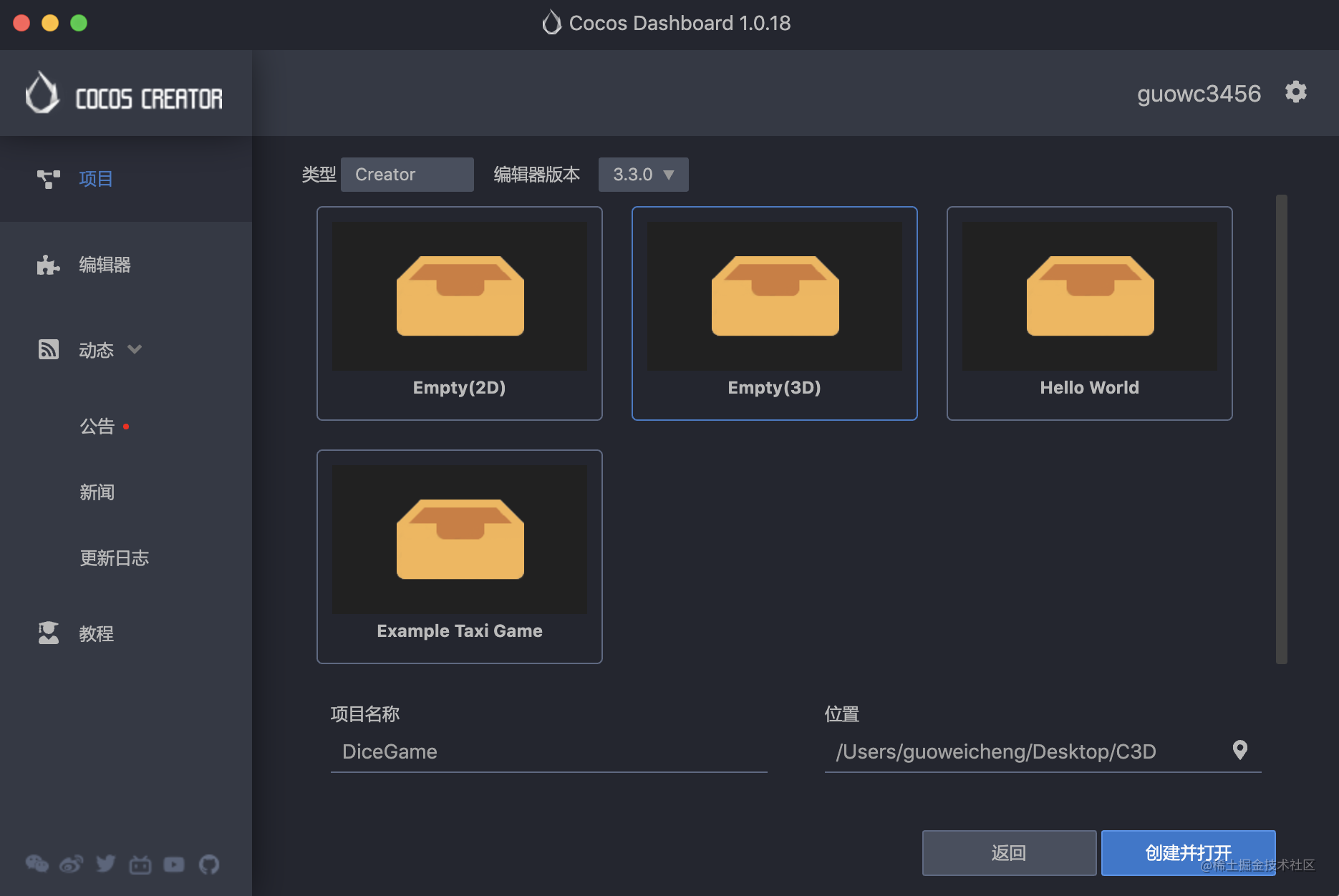## 素材资源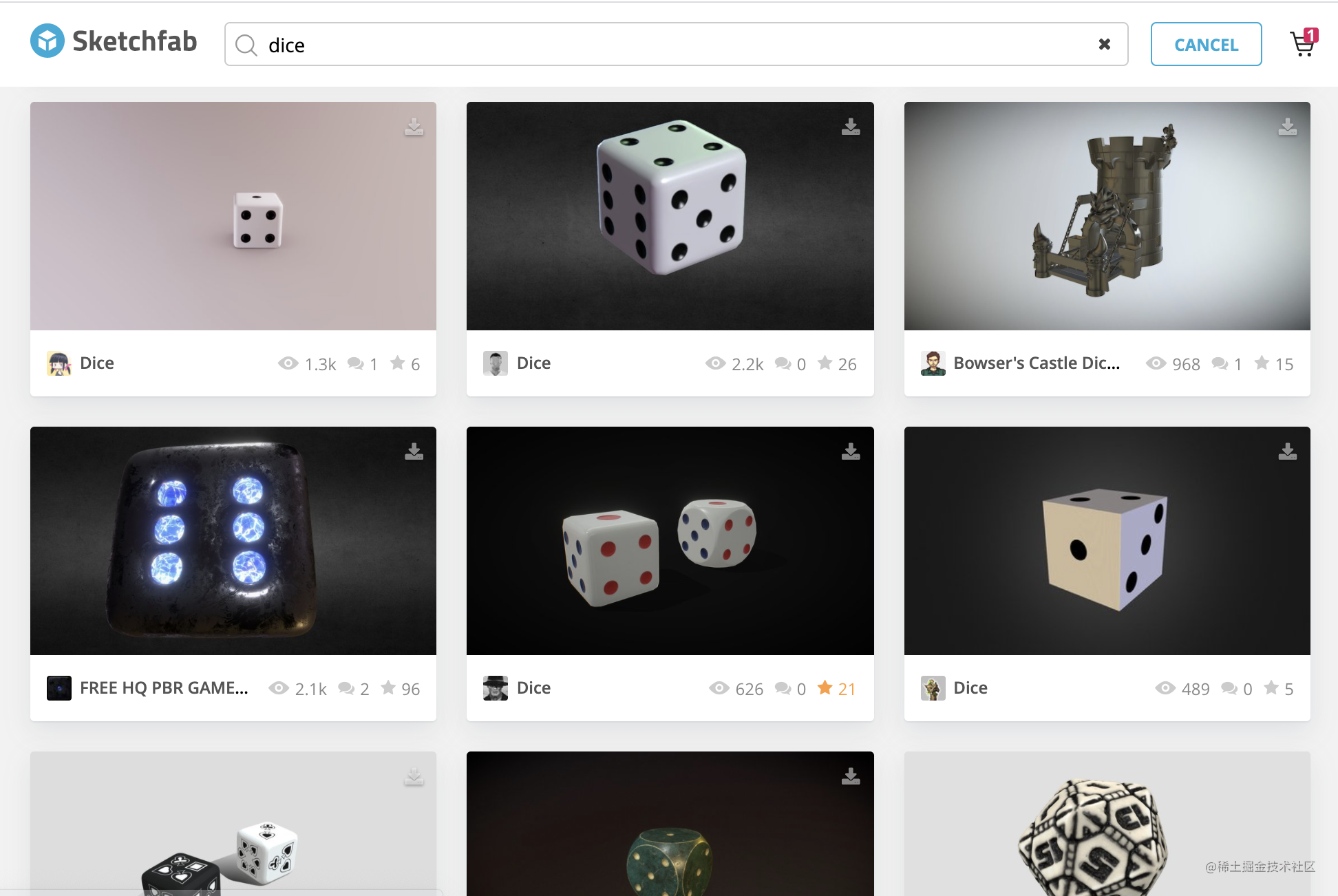CocosCreator目前支持GLTF/FBX两种格式，选择任意一种下载即可

## 音效资源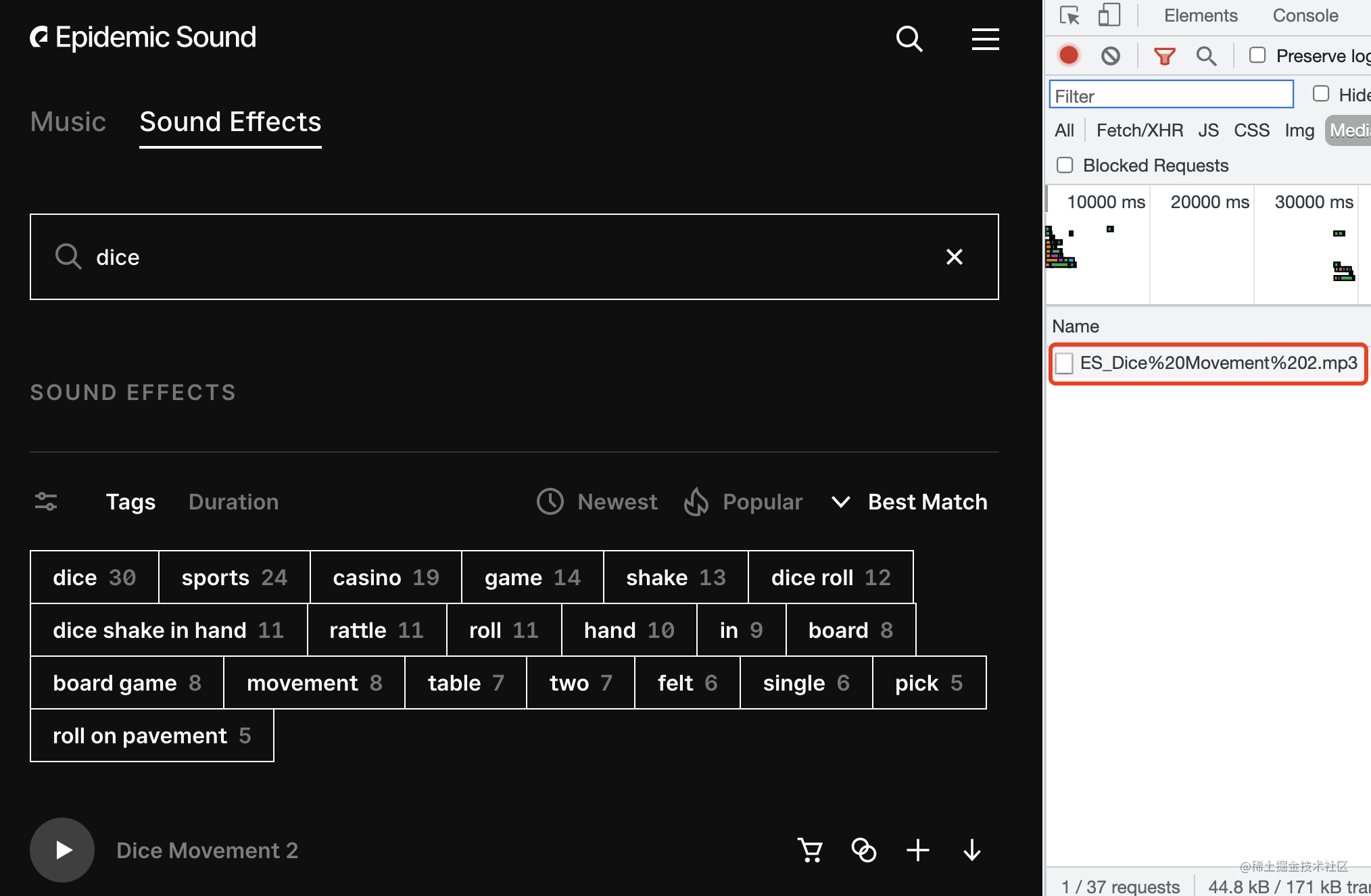# step.2 构建场景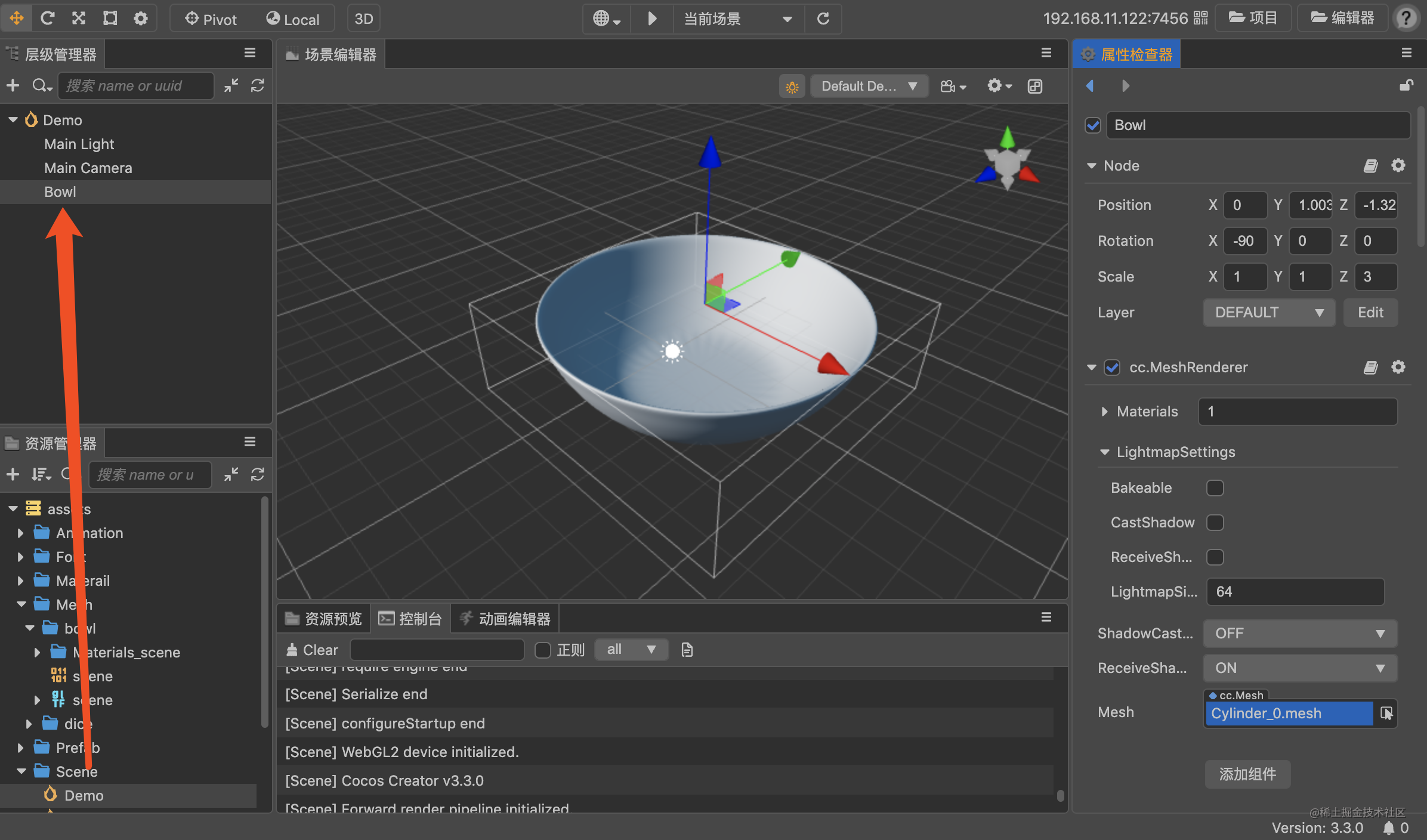右侧属性面板找到材质选项，调整材质的颜色，金属强度，粗糙度等，让模型更有质感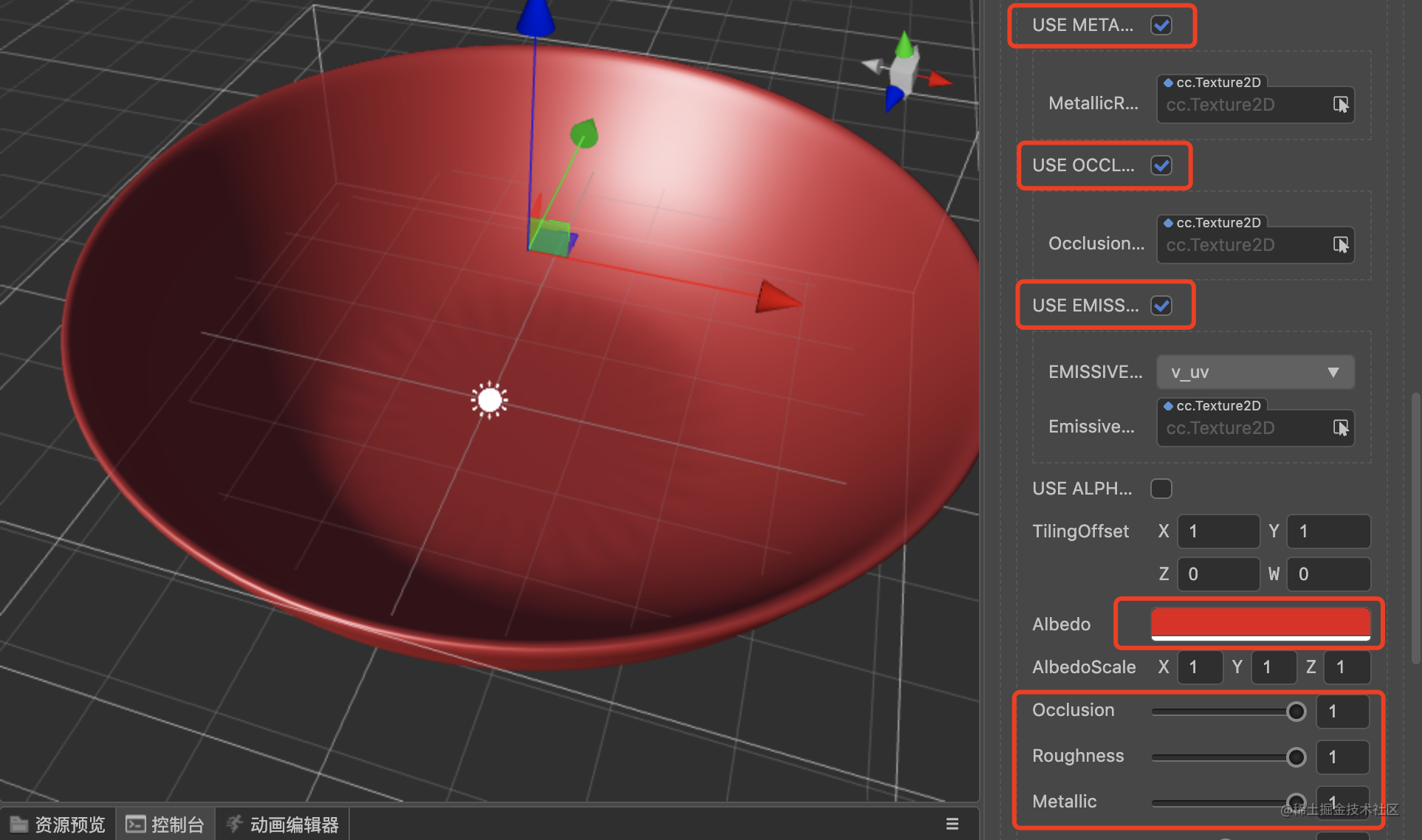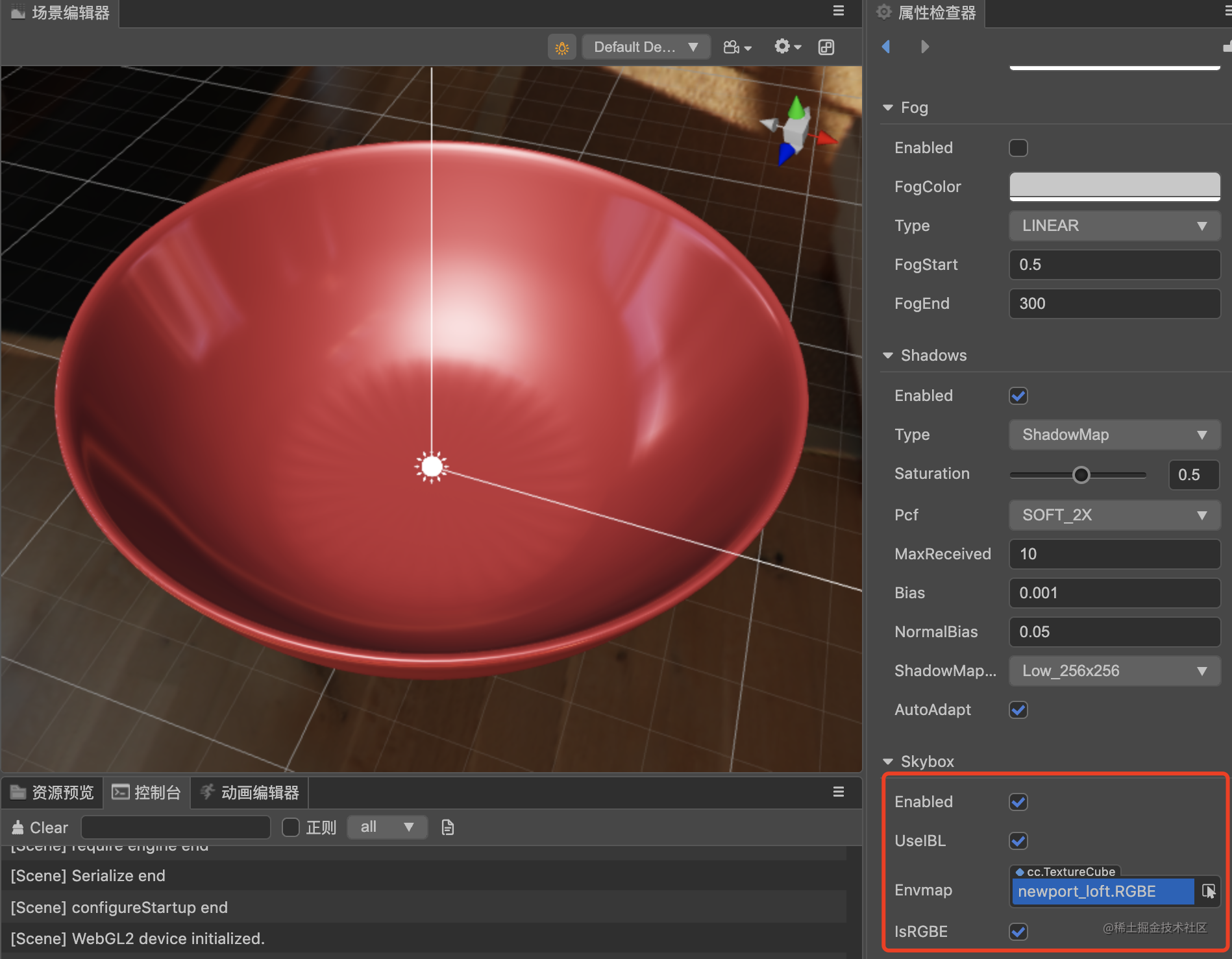天空盒资源：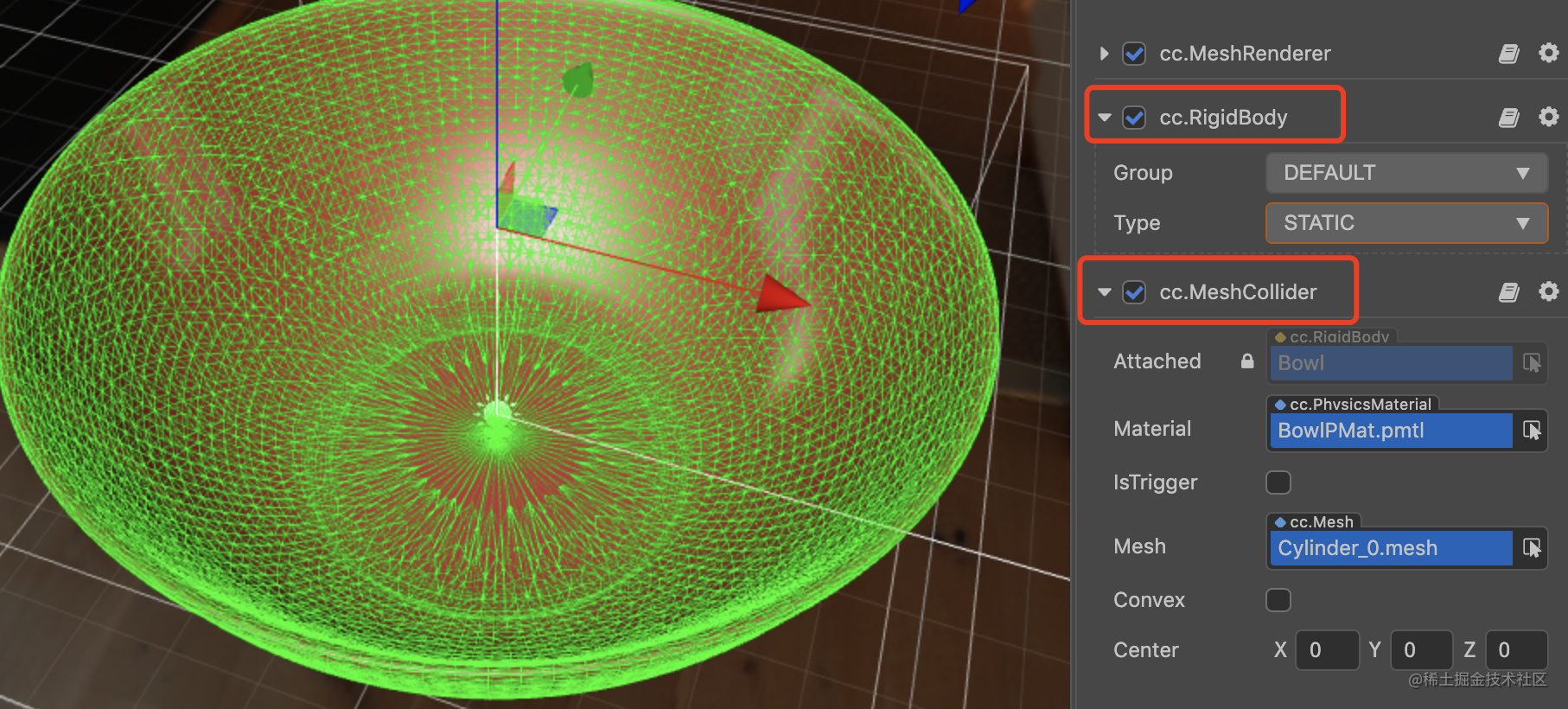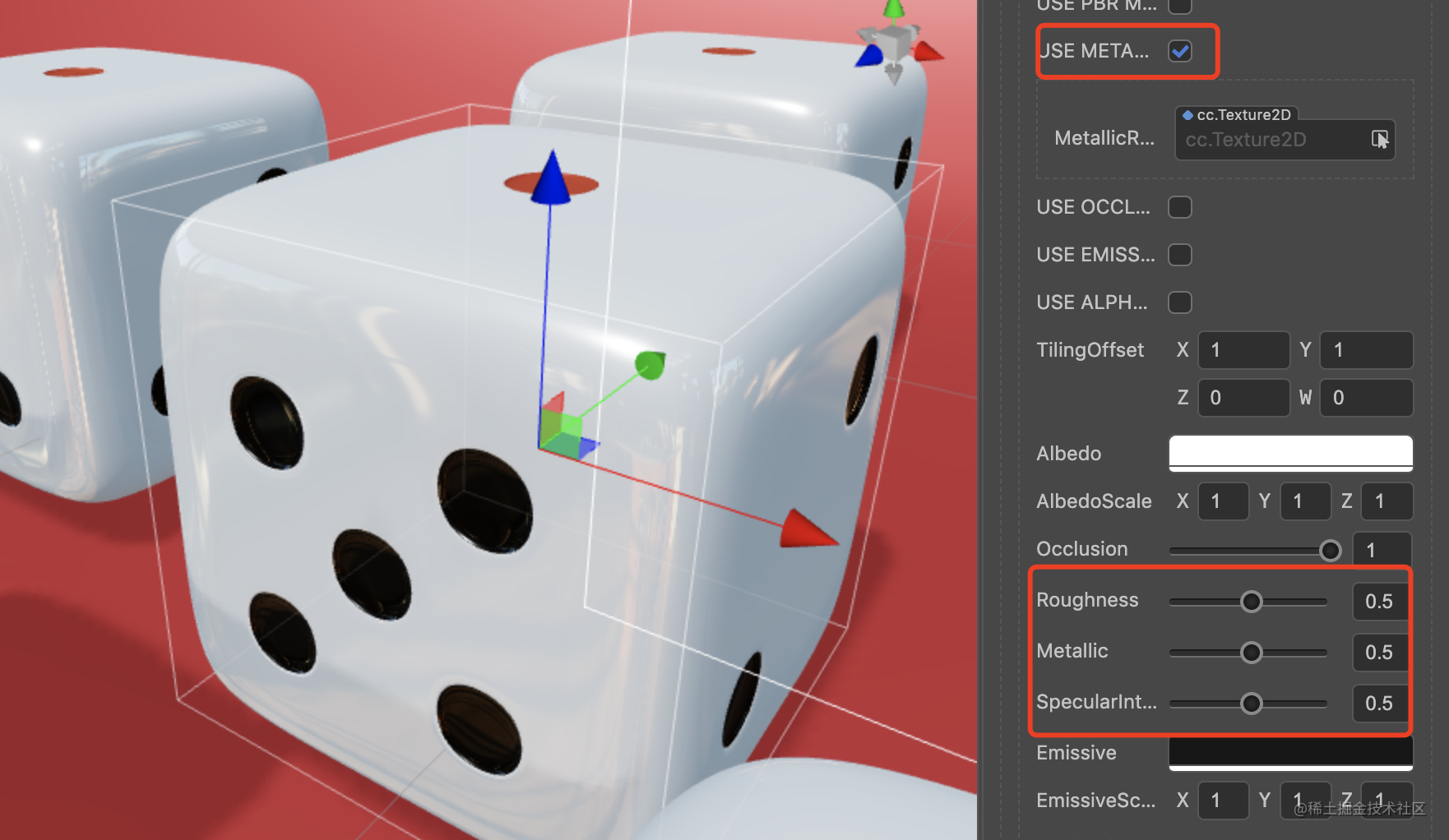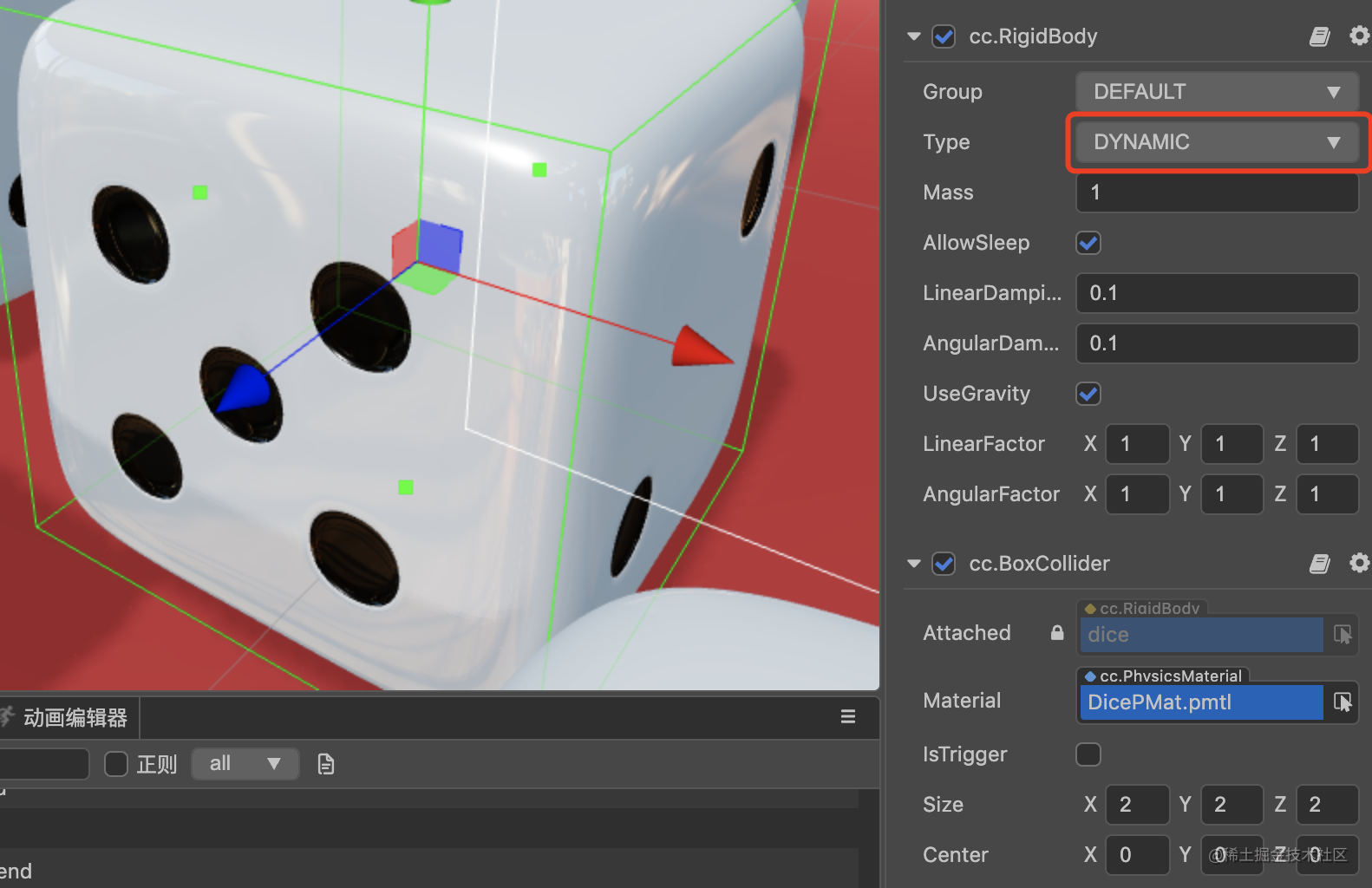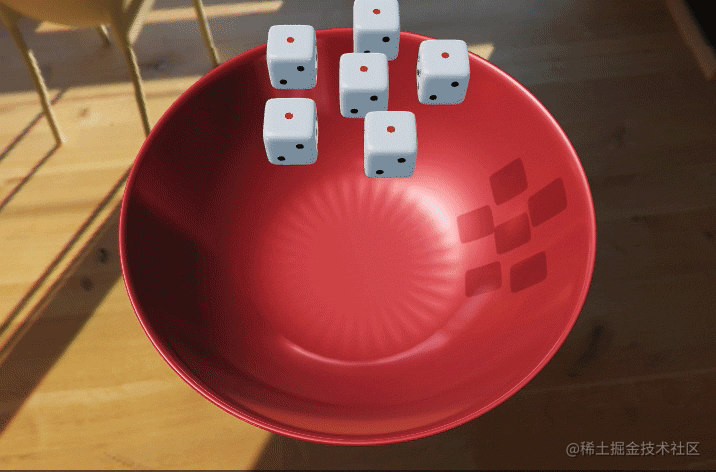# step.3 游戏逻辑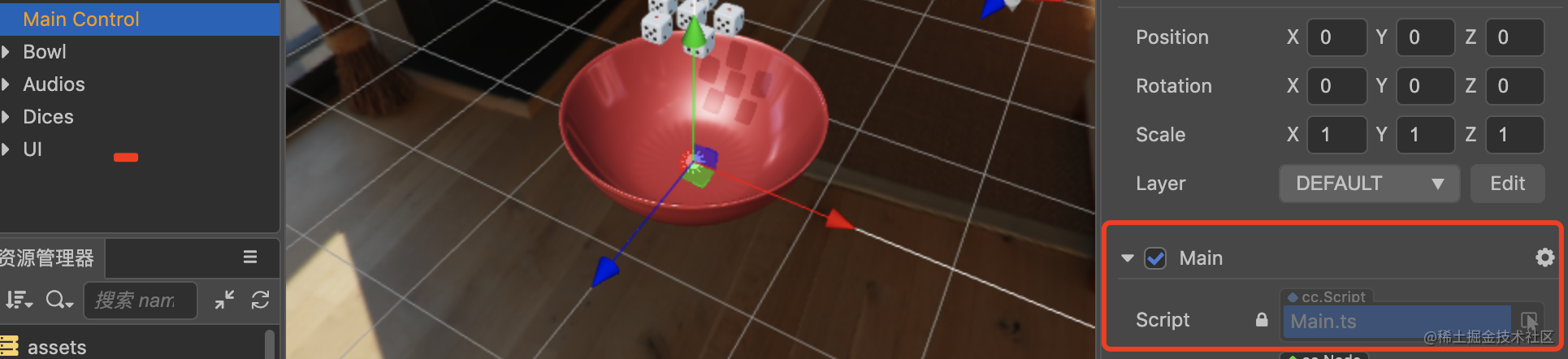``````@property(Node)
public dices: Node = null;
start () {
this.dice = this.dices.children; // 保存获取到的6个骰子节点
systemEvent.on(SystemEvent.EventType.TOUCH_START, this.onTouchStart, this);
systemEvent.on(SystemEvent.EventType.TOUCH_MOVE, this.onTouchMove, this);
systemEvent.on(SystemEvent.EventType.TOUCH_END, this.onTouchEnd, this);
}
onTouchStart (e) {
for (let i = 0; i < this.dice.length; i++) {
let dice = this.dice[i];
let rb = dice.getComponent(RigidBody); // 遍历骰子节点，获取节点上的刚体组件
rb.setLinearVelocity(new Vec3(0,0,0)); // 把刚体的速度设置为0
setTimeout(() => {
rb.type = ERigidBodyType.KINEMATIC;
// 把刚体类型设置为运动学刚体，动态刚体不能通过脚本干预运动方式，在操控骰子时要先把刚体类型设置为KINEMATIC
}, 0);
// 刚体类型修改在当前帧不生效，所以加了个setTimeout强制在下一帧执行
dice.setPosition(dice.position.x, 3, dice.position.z); //只改变Y的值，骰子定位到正上方
}
}
onTouchMove (e) {
// 拖拽骰子
var delta = e.getDelta();
for (var i = 0; i < this.dice.length; i++) {
let dice = this.dice[i];
dice.setPosition(dice.position.x - delta.y / 200, 3, dice.position.z - delta.x / 200);
}
}
onTouchEnd () {
// 释放骰子，并加上一个随机的速度和角速度冲量
for (var i = 0; i < this.dice.length; i++) {
let dice = this.dice[i];
let rb = dice.getComponent(RigidBody);
setTimeout(() => {
let r = (Math.random() - 0.5) * this.power;
let ry = -(Math.random() + 0.2) * this.power;
let rt = (Math.random() - 0.5) * this.power * 20;
rb.type = ERigidBodyType.DYNAMIC;  // 将RigidBody设置回动态
rb.applyImpulse(new Vec3(r, ry, r), new Vec3(0,0,0));
rb.applyTorque(new Vec3(rt, rt, rt));
}, 0);
}
}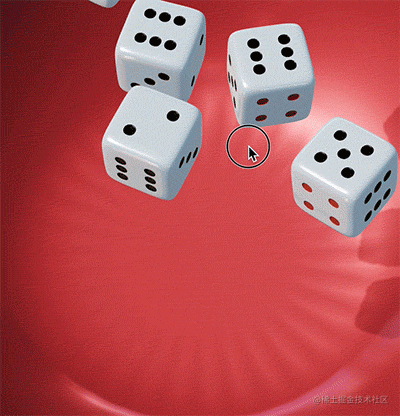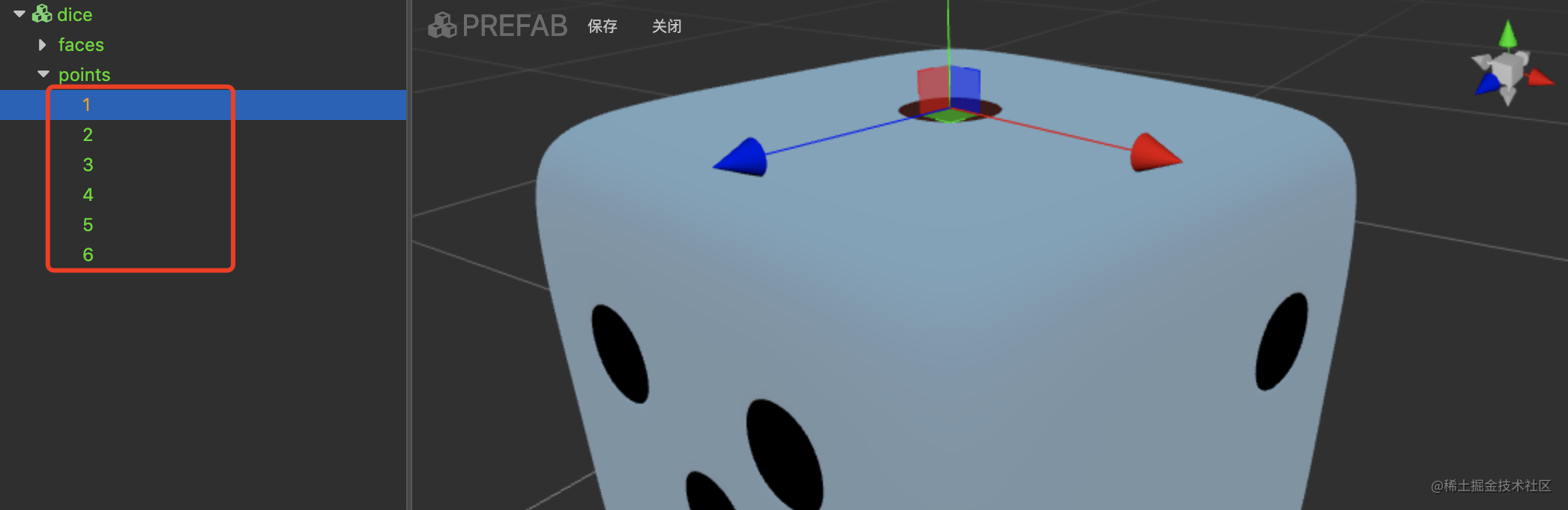# step.4 细节完善

``````start () {
this.rb = this.getComponent(RigidBody);
this.vel = new Vec3(0,0,0);
let Cld = this.getComponent(Collider);
Cld.on('onCollisionEnter', this.onCollision, this); // 碰撞事件监听
}
private onCollision (event: ICollisionEvent) {
let curTime = new Date().getTime();
if (curTime - this.lastTime > 50) {
// 过渡50ms内的连续碰撞
this.rb.getLinearVelocity(this.vel); // 获取碰撞时刚体的相对速度
this.audioSource.volume = Math.pow(Vec3.len(this.vel), 2) / 100; //根据相对速度设置音量
this.audioSource.play();
}
this.lastTime = curTime;
}

# final DEMO演示

H5版：Have fun!